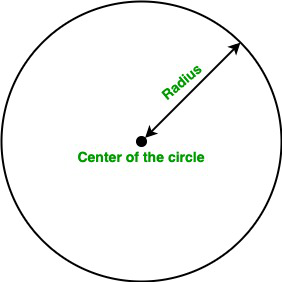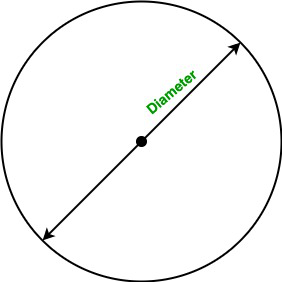Related Articles

• Last Updated : 04 Dec, 2020

A circle is a simple shape consisting of all the points in the plane that are equidistant from a point known as the center of the circle. In this article, we will learn how to find the area of the circle using the method overloading.

Terminology:

• Method Overloading: Method overloading allows different methods to have the same name, but different signatures where the signature can differ by the number of input parameters or type of input parameters or both.
• Area: A quantity that representhe extent of a 2-dimensional figure or shape in the plane is known as an area.
• Radius: The line segment from the center to any point of the circle is known as radius.
• Diameter: The line segment whose endpoints lie on the circle and passed through the center is known as the diameter of the circle. It is also known as the largest distance between any two points on the circle.

#### Area of the Circle

The area of the circle is the product of the square of the radius of the circle and the value of PI. We can simply calculate the area of the circle using the following formula:

• Using the radius of the circle:Formula:

Area of the circle:  A = π * r2

Here, r is the radius of the circle.

• Using the diameter of the circle:Formula:

Area of the circle:  A = (π / 4) * d2

Here, r is the radius of the circle.

Note: The value of PI in java is 3.141592653589793.

Below is the implementation of the above approach:

Example 1:

## Java

 `// Java program to find the area of``// the circle using Method Overloading`` ` `import` `java.io.*;`` ` `class` `Circle {`` ` `    ``static` `final` `double` `PI = Math.PI;`` ` `    ``// Overloaded Area() function to``    ``// calculate the area of the circle.``    ``// It takes one double parameter``    ``void` `Area(``double` `r)``    ``{``        ``double` `A = PI * r * r;`` ` `        ``System.out.println(``"Area of the circle is :"` `+ A);``    ``}`` ` `    ``// Overloaded Area() function to``    ``// calculate the area of the circle.``    ``// It takes one float parameter``    ``void` `Area(``float` `r)``    ``{``        ``double` `A = PI * r * r;`` ` `        ``System.out.println(``"Area of the circle is :"` `+ A);``    ``}``}`` ` `class` `GFG {`` ` `    ``// Driver code``    ``public` `static` `void` `main(String[] args)``    ``{`` ` `        ``// Creating object of Circle class``        ``Circle obj = ``new` `Circle();`` ` `        ``// Calling function``        ``obj.Area(``5``);``        ``obj.Area(``2.5``);``    ``}``}`
Output
```Area of the circle is :78.53981633974483
Area of the circle is :19.634954084936208```

Example 2:

## Java

 `// Java program to find the area of``// the circle when the diameter is given``// using Method Overloading`` ` `import` `java.io.*;`` ` `class` `Circle {`` ` `    ``static` `final` `double` `PI = Math.PI;`` ` `    ``// Overloaded Area() function to``    ``// calculate the area of the circle.``    ``// It takes one double parameter``    ``void` `Area(``double` `D)``    ``{``        ``double` `A = (PI / ``4``) * D * D;`` ` `        ``System.out.println(``"Area of the circle is :"` `+ A);``    ``}`` ` `    ``// Overloaded Area() function to``    ``// calculate the area of the circle.``    ``// It takes one float parameter``    ``void` `Area(``float` `D)``    ``{``        ``double` `A = (PI / ``4``) * D * D;`` ` `        ``System.out.println(``"Area of the circle is :"` `+ A);``    ``}``}`` ` `class` `GFG {`` ` `    ``// Driver code``    ``public` `static` `void` `main(String[] args)``    ``{`` ` `        ``// Creating object of Circle class``        ``Circle obj = ``new` `Circle();`` ` `        ``// Calling function``        ``obj.Area(``10``);``        ``obj.Area(``20.4``);``    ``}``}`
Output
```Area of the circle is :78.53981633974483
Area of the circle is :326.851299679482```

Attention reader! Don’t stop learning now. Get hold of all the important Java Foundation and Collections concepts with the Fundamentals of Java and Java Collections Course at a student-friendly price and become industry ready. To complete your preparation from learning a language to DS Algo and many more,  please refer Complete Interview Preparation Course.

My Personal Notes arrow_drop_up